##### 1 插入排序

•Sorting problem:

• Input: A sequence of n numbers a~1~, a~2~, …, a~n~
• Output: A permutation (reordering) a’~1~, a’~2~, …, a’~n~ of the input sequence such that

a’~1~<=a’~2~ <= … <=a’~n~

•An instance of the sorting problem

–Input: 8 2 4 9 3 6

–Output: 2 3 4 6 8 9

•Notation:

–Sorting is a fundamental operation in CS

–A large number of good sorting algorithms have been D&R

Worst-case: (usually)

–T(n) = maximum time of algorithm on any input of size n.

T(n)=maxI {T(n,I)}

–Time Complexity (Default )

Average-case: (sometimes)

–T(n) = expected time of algorithm over all inputs of size n.

–Need assumption of statistical distribution of inputs.

?

Best-case: (bogus虚假)

–Cheat with a slow algorithm that works fast on some input.

?
##### 2 时间复杂度描述

$$\Theta(n) \quad既有上界又有下界$$

Engineering:

–Drop low-order terms; ignore leading constants.

example:
$$3n^3 + 90n^2 –5n + 6046 = Θ(n3)$$

$$O(n)\quad 只有上界$$

$$Ω(n)\quad 只有下界$$

##### 3 分治思想

recursively 递归

subproblems 子问题

median 中位数

• Merge sort

•Problem:

–Input: A[1,n]

–Output: A[1,n] in sorted order

Divide: Divide the n-element sequence to be sorted into two subsequences of n/2 elements each.

Conquer: Sort the two subsequences recursively using merge sort.

Combine: Merge the two sorted subsequences to produce the sorted answer.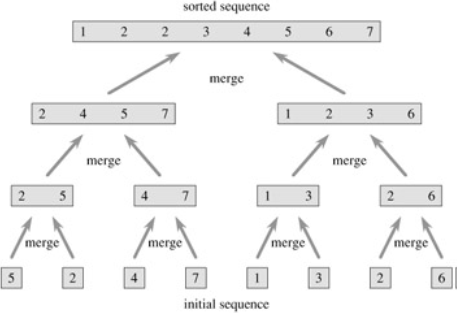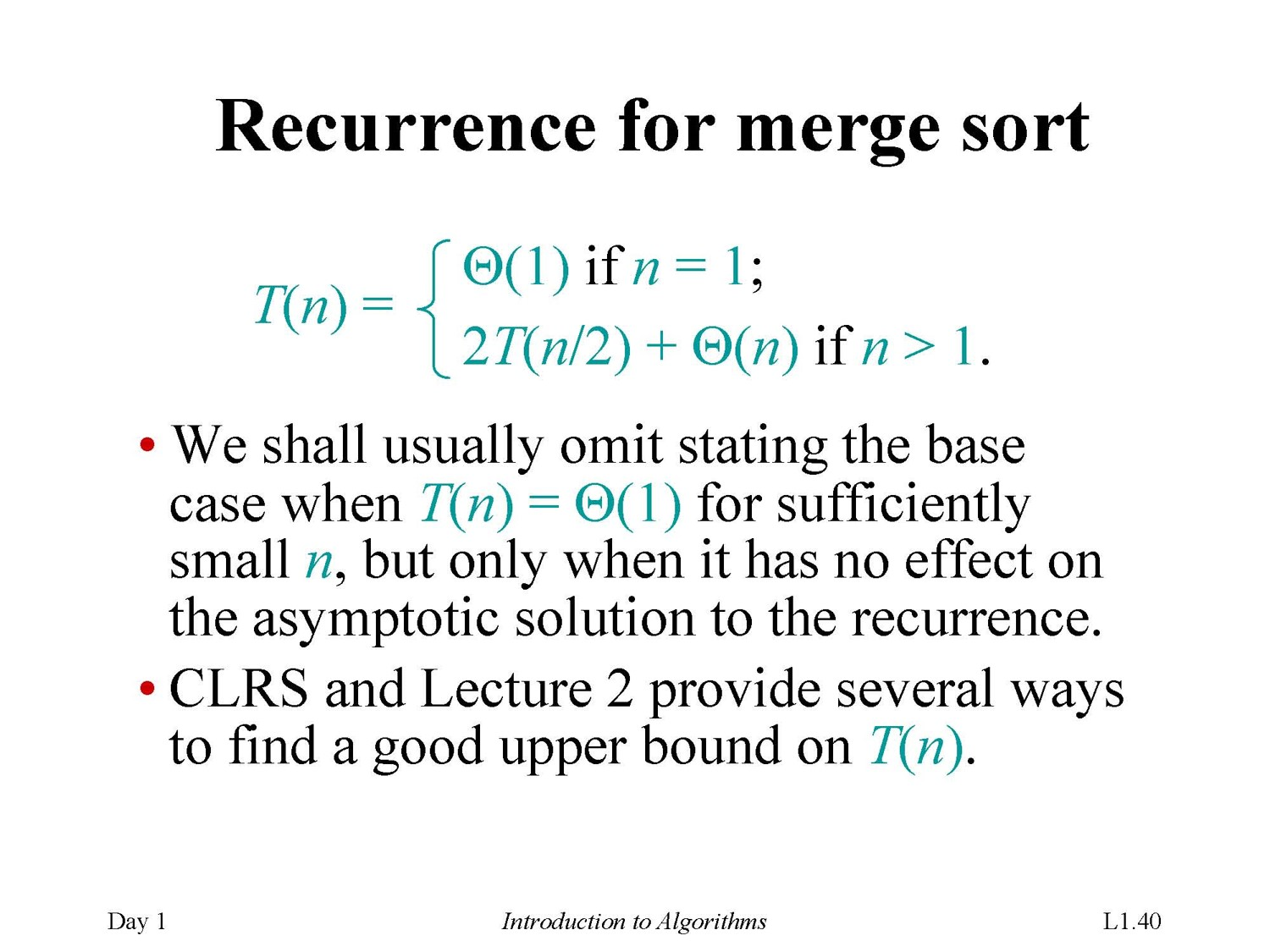• Recursion tree递归树解决递归式问题

例子
$$Solve \quad T(n)=2T(n)+cn,\quad where c>0\quad is \quad constant$$

##### 4 快速排序

​ 通过一趟排序将要排序的数据分割成独立的两部分，其中一部分的所有数据都比另外一部分的所有数据都要小，然后再按此方法对这两部分数据分别进行快速排序，整个排序过程可以递归进行，以此达到整个数据变成有序序列。

the run time drops from Ω(n log n) to Ω(n^2^)

Median-of-three(三平均分区法)

（1） 首先，它使得最坏情况发生的几率减小了。
（2） 其次，未改进的快速排序算法为了防止比较时数组越界，在最后要设置一个哨点。

##### 5 Dynamic programming 动态规划

Rod cutting

Given a rod of length n inches and a table of prices pi for i = 1,2,3,…,n, determine the maximum revenue(收入) r(n) obtainable by cutting up the rod and
selling the pieces.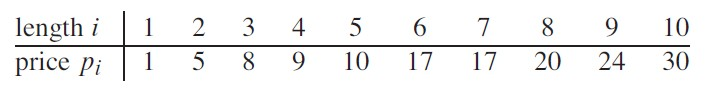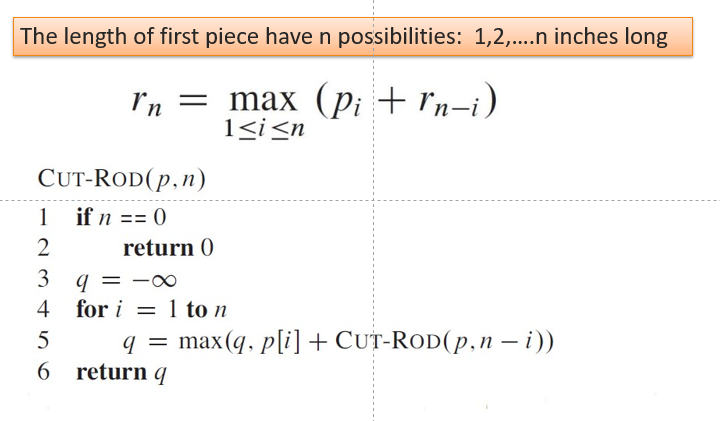##### 6 贪心算法

453 + 30 3 + 29 3 + 10 3 + 8 3 + 5 3 = 381个字符

EF最小，首先构造EF的生成树，重新排序

451 + 30 2 + 29 3 + 10 4 + 8 5 + 5 5 = 292个字符，节约了23%的成本！

End

##### 7 最大流问题

digraph G=(V,E)

weights, called capacities on edge c(u,v)边缘容量

two distinct vertices (不同的顶点)

source, ‘s’; sink ‘t’

The value of a flow is the net flow out of the source:
$$\sum p(s,v)-\sum p(v,s)$$

The value of this flow is 1-0+2=3

，有：

Rabin-KarpRabin-Karp

##### 8 字符串匹配

Rabin-Karp算法

p=P[m]+10(P[m-1]+10(P[m-2]+…+10(P+10P1)…)))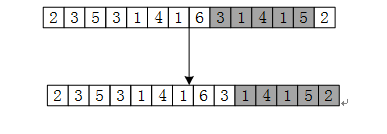ts+1=(ts-(T[s+1]=3)10^(m=4))10+(T[s+m+1]=2)

KMP算法

• 假设现在文本串S匹配到 i 位置，模式串P匹配到 j 位置

• 如果j = -1，或者当前字符匹配成功（即S[i] == P[j]），都令i++，j++，继续匹配下一个字符；
• 如果j != -1，且当前字符匹配失败（即S[i] != P[j]），则令 i 不变，j = next[j]。此举意味着失配时，模式串P相对于文本串S向右移动了j - next [j] 位。
• 换言之，当匹配失败时，模式串向右移动的位数为：失配字符所在位置 - 失配字符对应的next 值（next 数组的求解会在下文中详细阐述），即移动的实际位数为：j - next[j]，且此值大于等于1。

next数组的含义：代表当前字符之前的字符串中，有多大长度的相同前缀后缀，例如如果next[j]=k,代表j之前的字符串中有最大长度为k的相同前缀后缀。

• 对于P = p0 p1 …pj-1 pj，寻找模式串P中长度最大且相等的前缀和后缀。如果存在p0 p1 …pk-1 pk = pj- k pj-k+1…pj-1 pj，那么在包含pj的模式串中有最大长度为k+1的相同前缀后缀。举个例子，如果给定的模式串为“abab”，那么它的各个子串的前缀后缀的公共元素的最大长度如下表格所示：• next 数组考虑的是除当前字符外的最长相同前缀后缀，所以通过第①步骤求得各个前缀后缀的公共元素的最大长度后，只要稍作变形即可：将第①步骤中求得的值整体右移一位，然后初值赋为-1，如下表格所示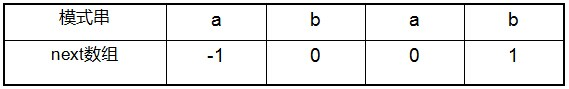kmp算法的复杂度是O(n+m)

https://blog.csdn.net/v_july_v/article/details/7041827#t5